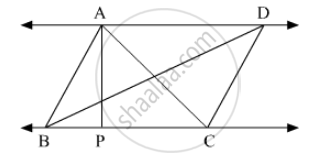# In following figure, AP ⊥ BC, AD || BC, then Find A(∆ABC): A(∆BCD). - Geometry

Sum

In the following figure, AP ⊥ BC, AD || BC, then Find A(∆ABC): A(∆BCD).#### Solution

Given, AP ⊥ BC, and AD || BC. ΔABC and ΔBCD has the same base BC.

Areas of triangles with equal bases are proportional to their corresponding heights.
Since AP is the perpendicular distance between parallel lines AD and BC, the height of ΔABC and the height of ΔBCD are the same.

∴ (A(ΔABC))/(A(ΔBCD)) = "AP"/"AP" = 1

Hence A(ΔABC): A(ΔBCD) = 1: 1

Concept: Similar Triangles
Is there an error in this question or solution?

#### APPEARS IN

Balbharati Mathematics 2 Geometry 10th Standard SSC Maharashtra State Board
Chapter 1 Similarity
Practice Set 1.1 | Q 4 | Page 6